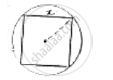Share

# If a Square is Inscribed in a Circle, Find the Ratio of Areas of the Circle and the Square. - CBSE Class 10 - Mathematics

ConceptAreas of Combinations of Plane Figures

#### Question

If a square is inscribed in a circle, find the ratio of areas of the circle and the square.

#### SolutionLet side of square be x cms inscribed in a circle.

Radius of circle (r) =1/2(𝑑𝑖𝑎𝑔𝑜𝑛𝑎𝑙 𝑜𝑓 𝑠𝑞𝑢𝑎𝑟𝑒)

=1/2(sqrt(2x))

=x/sqrt(2)

Area of square = (side)2 = x2

Area of circle = 𝜋r2

=pi( x/sqrt(2))^2

=(pix^2)/2

"Area of circle"/"Area of square"=(pi/2x^2)/x^2=pi/2=pi:2

Is there an error in this question or solution?

#### APPEARS IN

Solution If a Square is Inscribed in a Circle, Find the Ratio of Areas of the Circle and the Square. Concept: Areas of Combinations of Plane Figures.
S